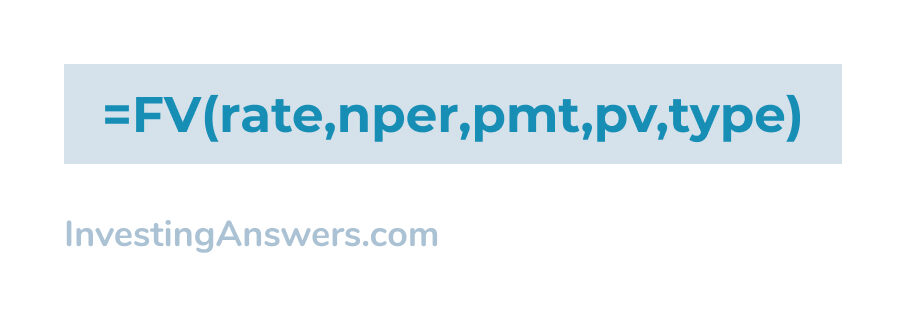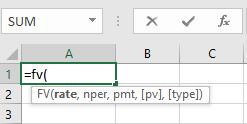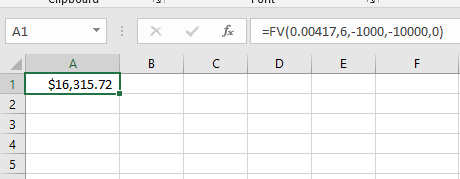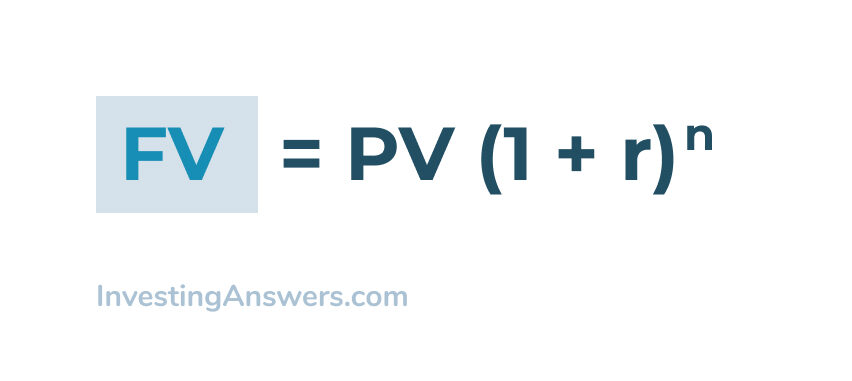Future value helps you project the value of your investments over a period of time. This is a basic time value of money calculation that is the basis for many financial investment strategies.

This will let you know if your current assumptions or activities will meet your financial goals.

Keep in mind, to do these calculations on a calculator, you’ll need a financial calculator. A regular one, or even a scientific calculator won’t have the functions you need to do this math.

## What Is Future Value?

Future value is the calculation of the value of your current financial holdings over time, using the expected interest rate or rate of growth. It tells you how much money you will have in the future.

### Example of Calculating Future Value

Let’s assume you’re trying to save up enough money to buy a car at the end of six months. You already have \$10,000 in an account that pays 5% interest per year. You’re able add \$1,000 to the account each month.

The value of the account in six months will be \$16,315.47. Here’s how we calculated this.

## How to Calculate Future Value On a Financial Calculator

To calculate future value on a financial calculator, you will need to enter in the following values:

• Number of Periods
• Starting Amount
• Interest Rate
• Periodic Deposit Amount

Here’s how to do this on a financial calculator:

### 1. Clear the Financial Calculator

Before we start, clear the financial keys by pressing [2nd] and then pressing [FV]. This will set the calculation up for future value. Since we have monthly payments, you should do everything in terms of months.

### 2. Enter The Number of Payments

This will be the number of periods involved in the calculation, which is the number of payments, which in this case is 6 months. On the calculator, press [N] and enter 6.

### 3. Enter Periodic Interest Rate

In our example, we are assuming a 5% APR interest rate. Using the monthly rate, 5%/12, enter this into the calculator by pressing I/YR and 5/12. This will auto-calculate your interest over a 1-year period.

### 4. Enter Payment Amount for Each Month

Enter the payment amount for each month by keying -\$1000 and pressing [PMT].

Note: Payment amounts are represented as a negative value because the payments represent cash outflows with respect to the investor.

### 5. Enter Present Value Amount

Enter the present value amount of -\$10,000 and press the [PV] key.

Note: Present value amounts are represented as a negative value because the payments represent cash outflows with respect to the investor.

### 6. Solve for Future Value On The Financial Calculator

To calculate FV, simply press the [CPT] key and then [FV]. Your answer should be exactly \$16,315.47.

If you're off by a few cents, it is probably because you used fewer decimal places in your periodic interest rate.

## How to Calculate Future Value in Excel

Another way to calculate future value is to enter your variables into an Excel spreadsheet. Here are the steps to build your calculation.

### Excel Formula for Future Value

Here is the formula used in Excel to calculate future value:Here's what each value means:

rate - periodic interest rate
nper - number of periods
pmt - payment made per period
pv - present value
type - when the payment is due (usually 'beginning of the month')

### 1. Calculate the Periodic Interest Rate

If you are calculating interest using annual percentage yield (APY), you will need to adjust your interest rate to match your period. If you want to break it down into months or days, you will need to divide the interest rate accordingly.

For our example, we are calculating the future value after 6 months. We will divide our 5% APY interest rate by 12 month, giving us 0.417% interest per month.

### 2. Fill in Cell Information

In Excel, start by entering the future value formula by keying in =FV(

Then enter each variable in the proper order:

rate - 0.417% interest per month
nper - 6
pmt - \$1,000
pv - \$10,000
type - enter '0' for calculating at the beginning of the month

###3. Calculate Future Value

Here’s what the complete formula will look like when all the variables are entered:

=FV(0.00417,6,-1000,-10000,0)

Now press [Enter] to automatically calculate the future value.

Using our example number, the total future value after 6 months should be \$16,315.72.Note: The calculations between Excel and the financial calculator method will vary slightly, depending on how many decimal points you calculate.

## Future Value Formula

When calculating the future value of an investment, here is the formula to use:Here's what each value means:

PV= Present value of the investment

FV = Future value of the investment

r = Interest rate

n = Number of time periods

### Example of Calculating Future Value

Putting this formula into practice, here is an example of finding the future value of your money:

Let’s assume you have \$10,000 in an account that pays 5% interest per year. If you want to know what that \$10,000 will be worth in six months, you can apply the future value formula.

FV = \$10,000 (1 + 0.05)0.5 = \$10,246.95

Your initial \$10,000 will grow by \$246.95 over a six-month period, assuming a constant 5% interest rate.